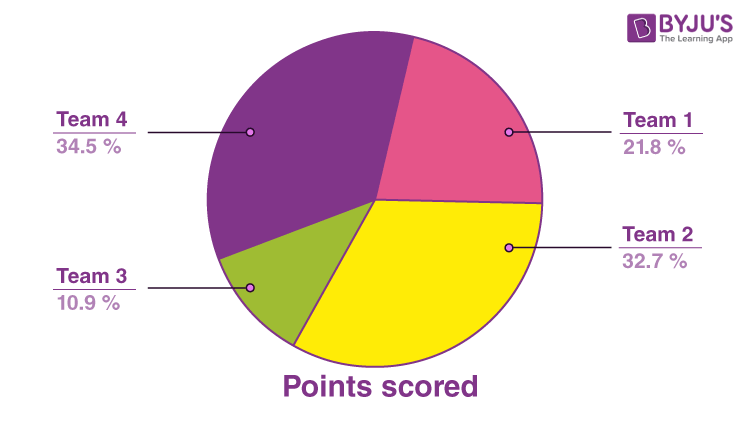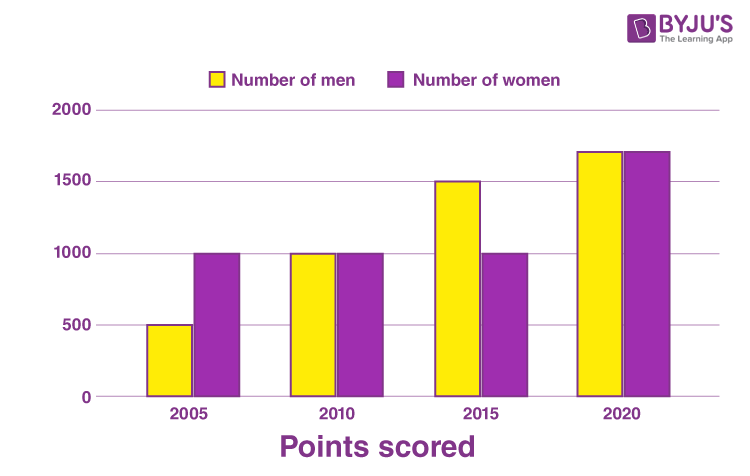Checkout JEE MAINS 2022 Question Paper Analysis : Checkout JEE MAINS 2022 Question Paper Analysis :

# Class 8 Maths Chapter 15 Introduction to Graphs MCQs

Class 8 Maths Chapter 15 Introduction to graphs MCQs (Questions and Answers) are available online for students, with detailed explanations. These MCQs are as per the latest exam pattern. The multiple-choice questions are designed by experts, according to the CBSE syllabus (2021-2022) and NCERT guidelines. The objective questions, chapter-wise, are given at BYJU’S to make students understand each concept and score better marks in exams. Also, learn important questions for class 8 Maths here.

Practice more and test your skills on Class 8 Maths Chapter 15 Introduction to graphs  MCQs with the given PDF here.

## MCQs Questions on Class 8 Chapter 15 Introduction to graphs

Multiple choice questions (MCQs) are given for the Class 8 Introduction to graphs chapter.

1. A ________ is a bar graph that shows data in intervals.

A. Bar-graph

B. Pie-chart

C. Histograph

D. Line Graph

2. A graph that displays data that changes continuously over periods of time is called:

A. Bar-graph

B. Pie-chart

C. Histograph

D. Line Graph

3. A line graph which is a whole unbroken line is called a:

A. Linear graph

B. Pie-chart

C. Histograph

D. Bar-graph

4. Which point lies only on y-axis?

A. (-2,0)

B. (2,0)

C. (0,-2)

D. (2,-2)

5. If we join (-3,2), (-3,-3) and (-3,4), then we obtain:

A. A triangle

B. Straight-line without passing through origin

C. Straight-line passing through origin

D. None of the above

6. The point (4,0) lies on which of the following?

A. x-axis

B. y-axis

C. origin

D. None of the above

7. The point (-2,-2) is:

A. near to x-axis

B. near to y-axis

C. near to origin

D. Equidistant from x-axis and y-axis.

8. The point (-2, 5) is nearer to:

A. x-axis

B. y-axis

C. origin

D. None of the above

9. The point (-5, 2) is nearer to:

A. x-axis

B. y-axis

C. origin

D. None of the above

10. The point (0,0) lies at:

A. x-axis

B. y-axis

C. origin

D. None of the above

Observe the diagram, given below and find the correct answer to the following MCQs.11. Which team has the highest score?

A. Team 1
B. Team 2
C. Team 3
D. Team 4

Explanation: Team 4 has the highest score with 34.5%.

12. Which team has the lowest score?

A. Team 1
B. Team 2
C. Team 3
D. Team 4

Explanation: Team 3 has the lowest score with 10.9%.

13. Which team is coloured green?

A. Team 1
B. Team 2
C. Team 3
D. Team 4

14. What is the average score of all the teams?

A. 22%
B. 25%
C. 27%
D. 29%

Explanation: Average score = (sum of all scores)/(total number of scores)

15. Which team has the second-highest score?

A. Team 1
B. Team 2
C. Team 3
D. Team 4

Explanation: Team 2 has the second-highest score, equal to 32.7%.

Below is the data of the number of men and women in a village for different years. Now based on this data answer the following MCQs with the correct option.16. How many men were there in the village in 2010?

A. 500
B. 1000
C. 1500
D. 2000

Explanation: There were 1000 men in the year 2010 in the village.

17. The population of men and women in 2020 is the same?

A. True
B. False

18. In which year the population of women is the highest?

A. 2005
B. 2010
C. 2015
D. 2020

19. When is the population of men, the minimum?

A. 2005
B. 2010
C. 2015
D. 2020

Explanation: In 2005, there were only 500 men in the village.

20. In which year population of men is more than women?
A. 2005
B. 2010
C. 2015
D. 2020